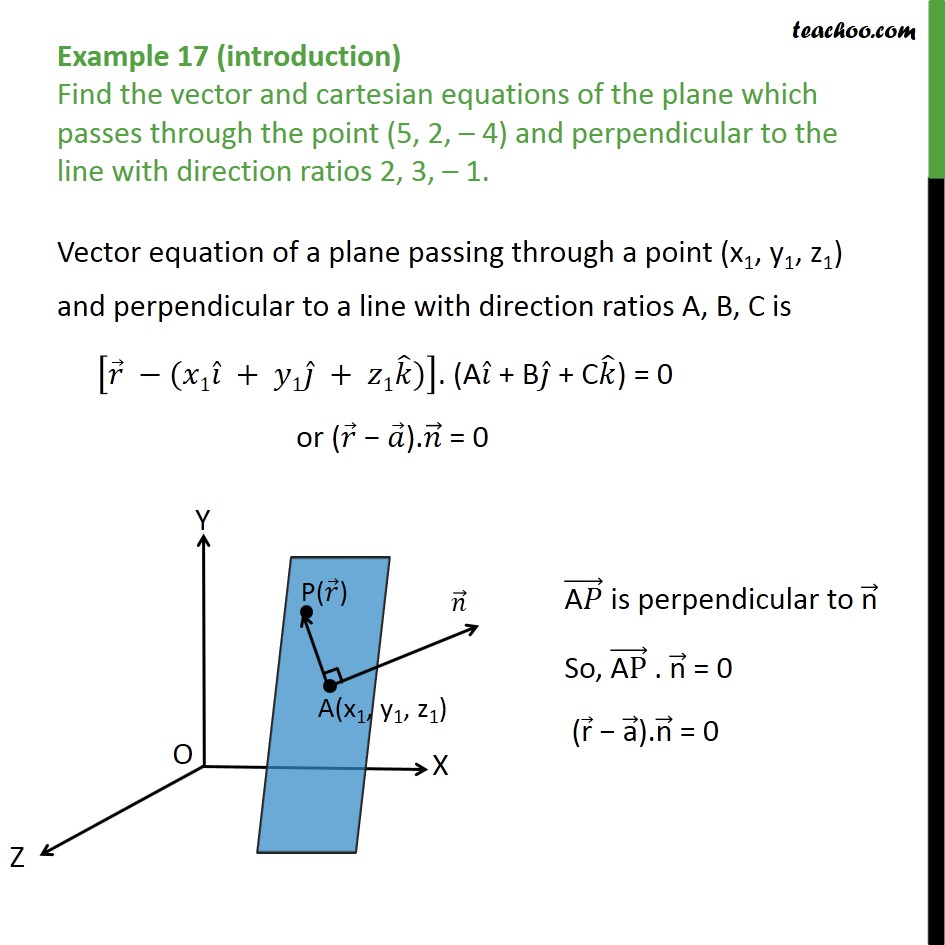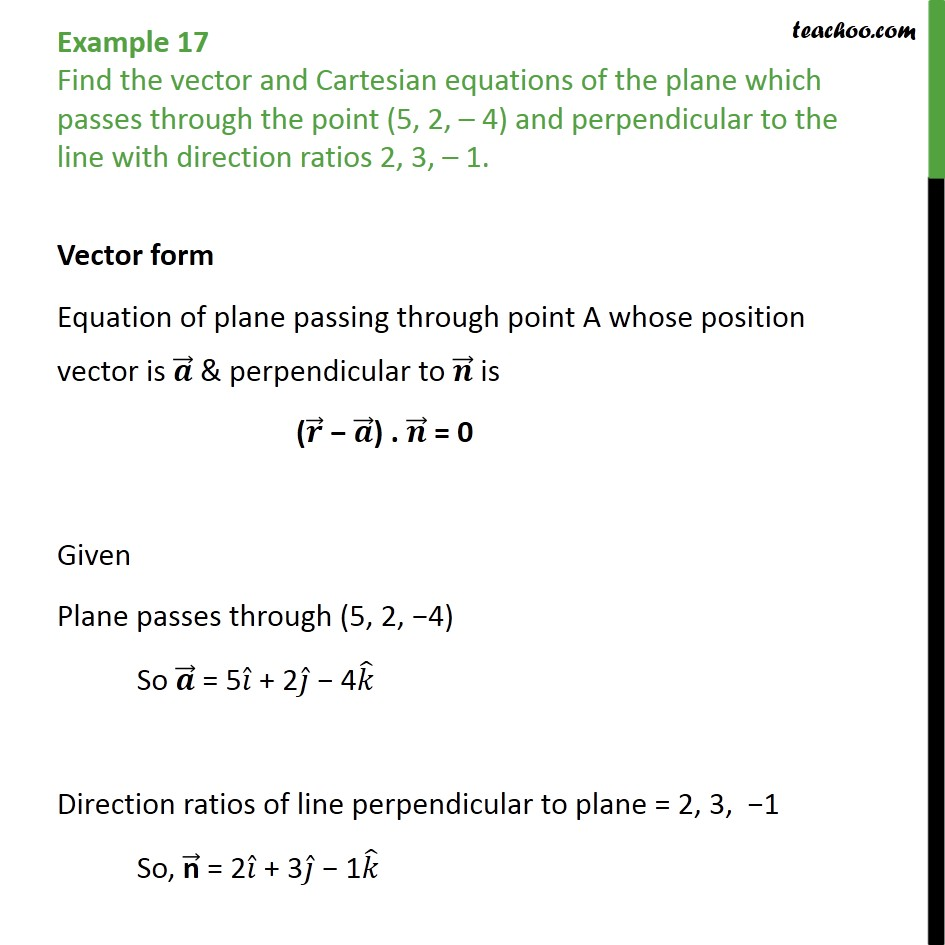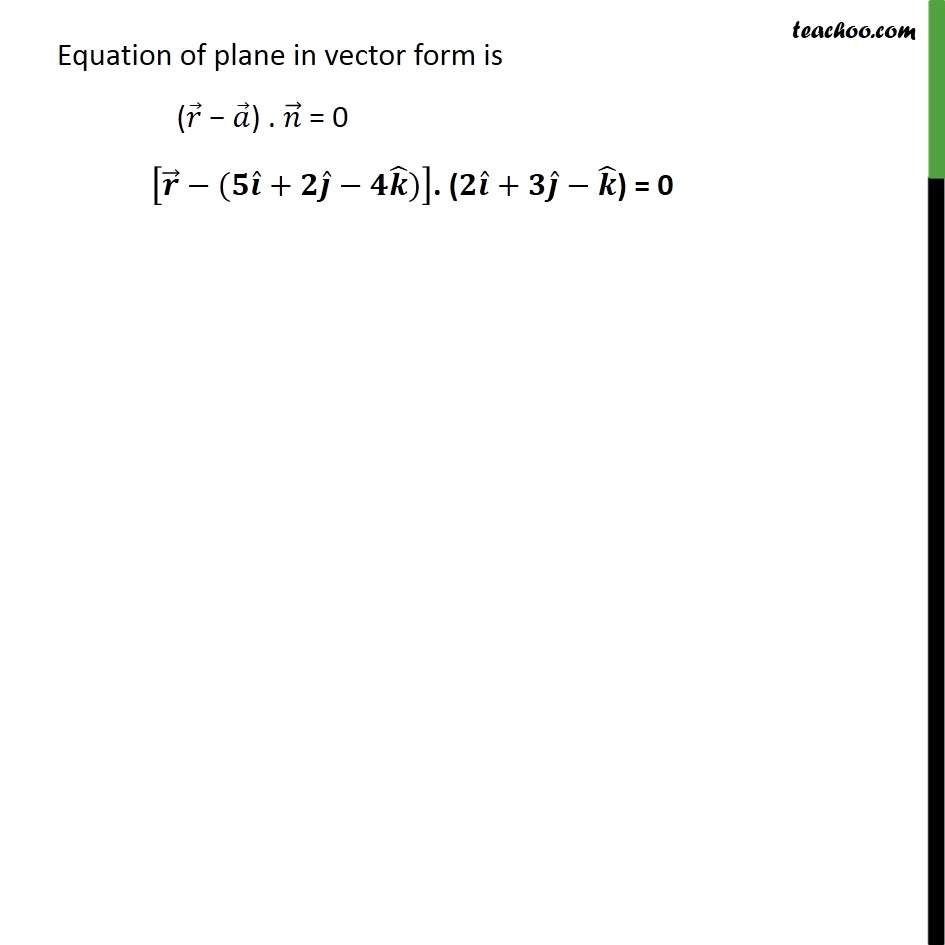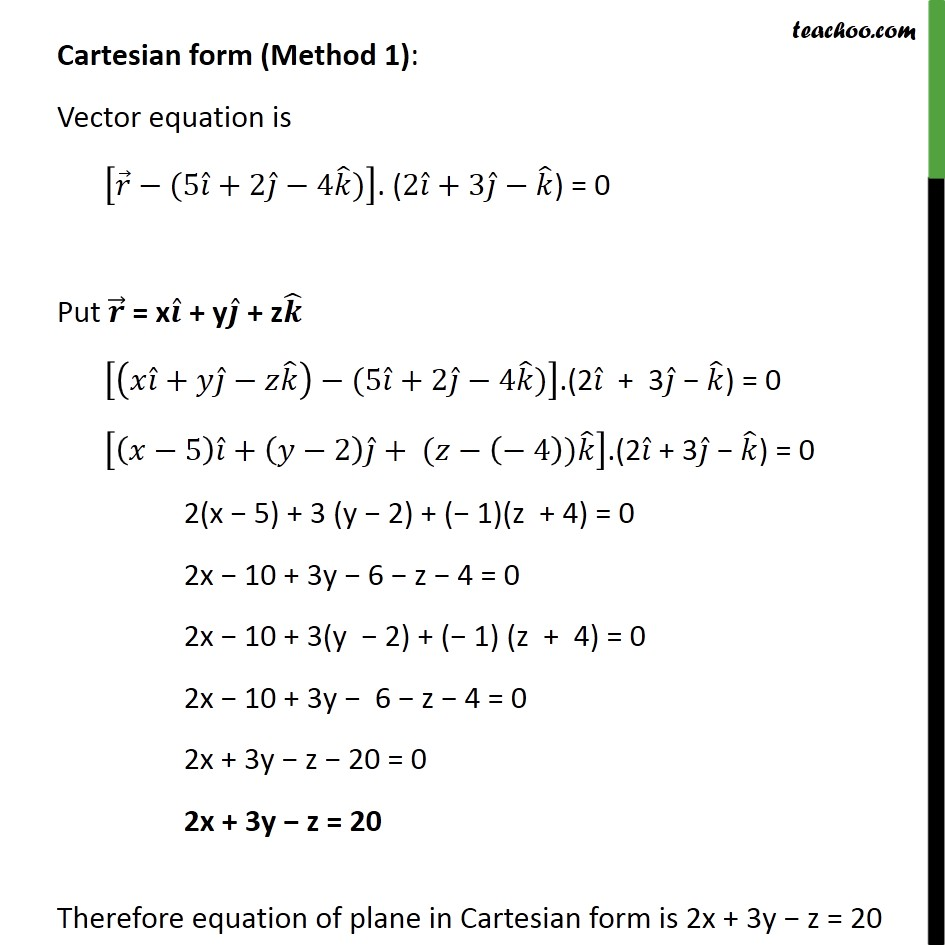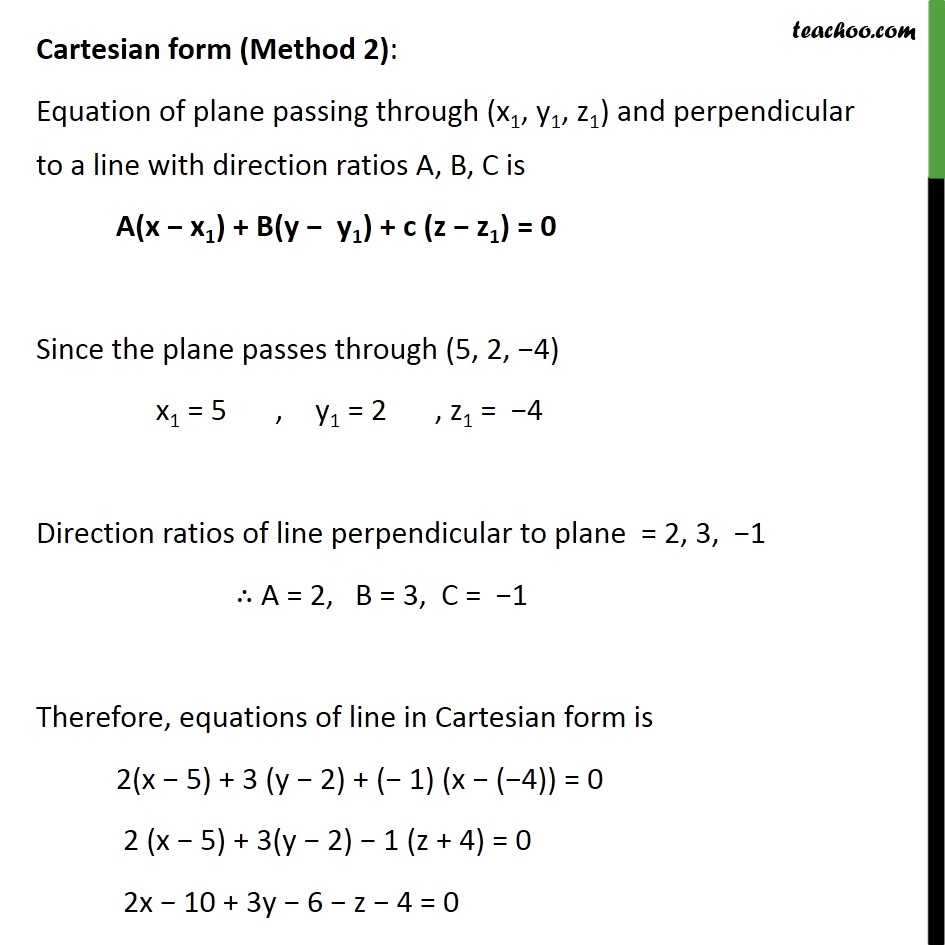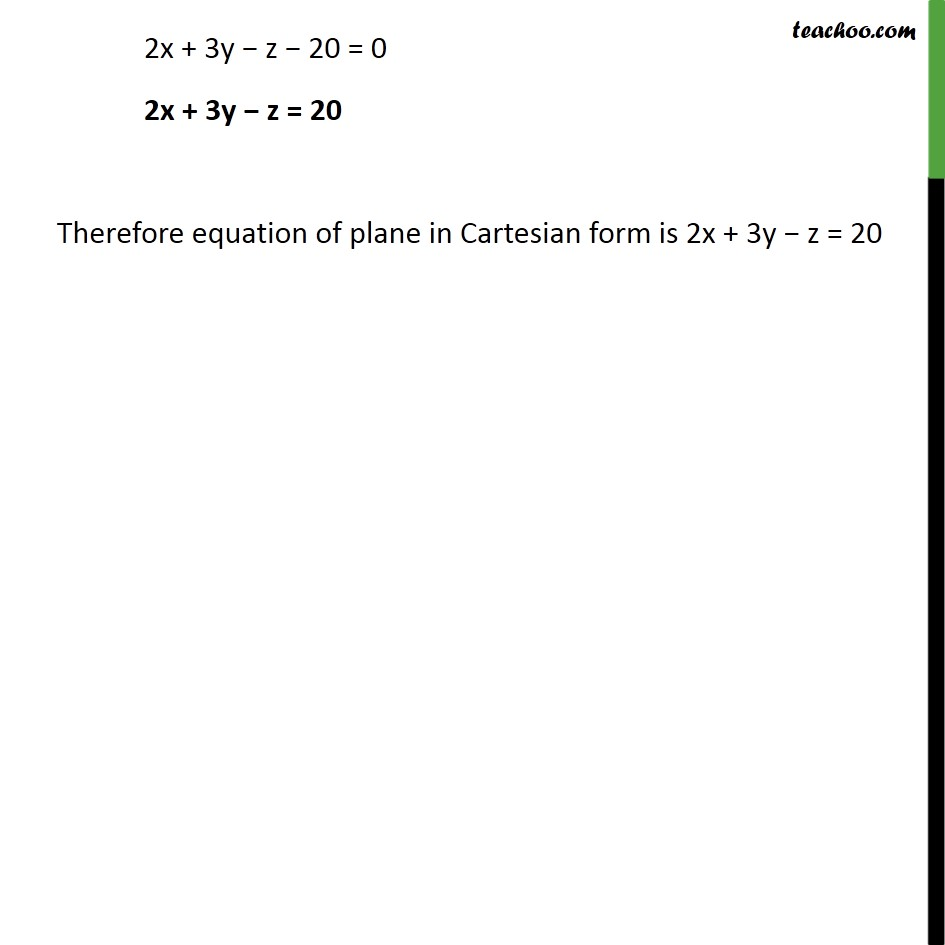1. Chapter 11 Class 12 Three Dimensional Geometry
2. Concept wise
3. Equation of plane - Prependicular to Vector & Passing Through Point

Transcript

Example 17 (introduction) Find the vector and cartesian equations of the plane which passes through the point (5, 2, – 4) and perpendicular to the line with direction ratios 2, 3, – 1. Vector equation of a plane passing through a point (x1, y1, z1) and perpendicular to a line with direction ratios A, B, C is 𝑟﷯ −(𝑥1 𝑖﷯ + 𝑦1 𝑗﷯ + 𝑧1 𝑘﷯)﷯. (A 𝑖﷯ + B 𝑗﷯ + C 𝑘﷯) = 0 or ( 𝑟﷯ − 𝑎﷯). 𝑛﷯ = 0 Example 17 Find the vector and Cartesian equations of the plane which passes through the point (5, 2, – 4) and perpendicular to the line with direction ratios 2, 3, – 1. Vector form Equation of plane passing through point A whose position vector is 𝒂﷯ & perpendicular to 𝒏﷯ is ( 𝒓﷯ − 𝒂﷯) . 𝒏﷯ = 0 Given Plane passes through (5, 2, −4) So 𝒂﷯ = 5 𝑖﷯ + 2 𝑗﷯ − 4 𝑘﷯ Direction ratios of line perpendicular to plane = 2, 3, −1 So, n﷯ = 2 𝑖﷯ + 3 𝑗﷯ − 1 𝑘﷯ Equation of plane in vector form is ( 𝑟﷯ − 𝑎﷯) . 𝑛﷯ = 0 𝒓﷯−(𝟓 𝒊﷯+𝟐 𝒋﷯−𝟒 𝒌﷯)﷯. (𝟐 𝒊﷯+𝟑 𝒋﷯− 𝒌﷯) = 0 Cartesian form (Method 1): Vector equation is 𝑟﷯−(5 𝑖﷯+2 𝑗﷯−4 𝑘﷯)﷯. (2 𝑖﷯+3 𝑗﷯− 𝑘﷯) = 0 Put 𝒓﷯ = x 𝒊﷯ + y 𝒋﷯ + z 𝒌﷯ 𝑥 𝑖﷯+𝑦 𝑗﷯−𝑧 𝑘﷯﷯−(5 𝑖﷯+2 𝑗﷯−4 𝑘﷯)﷯.(2 𝑖﷯ + 3 𝑗﷯ − 𝑘﷯) = 0 𝑥−5﷯ 𝑖﷯+ 𝑦−2﷯ 𝑗﷯+ (𝑧− − 4﷯) 𝑘﷯﷯.(2 𝑖﷯ + 3 𝑗﷯ − 𝑘﷯) = 0 2(x − 5) + 3 (y − 2) + (− 1)(z + 4) = 0 2x − 10 + 3y − 6 − z − 4 = 0 2x − 10 + 3(y − 2) + (− 1) (z + 4) = 0 2x − 10 + 3y − 6 − z − 4 = 0 2x + 3y − z − 20 = 0 2x + 3y − z = 20 Therefore equation of plane in Cartesian form is 2x + 3y − z = 20 Cartesian form (Method 2): Equation of plane passing through (x1, y1, z1) and perpendicular to a line with direction ratios A, B, C is A(x − x1) + B(y − y1) + c (z − z1) = 0 Since the plane passes through (5, 2, −4) x1 = 5 , y1 = 2 , z1 = −4 Direction ratios of line perpendicular to plane = 2, 3, −1 ∴ A = 2, B = 3, C = −1 Therefore, equations of line in Cartesian form is 2(x − 5) + 3 (y − 2) + (− 1) (x − (−4)) = 0 2 (x − 5) + 3(y − 2) − 1 (z + 4) = 0 2x − 10 + 3y − 6 − z − 4 = 0 2x + 3y − z − 20 = 0 2x + 3y − z = 20 Therefore equation of plane in Cartesian form is 2x + 3y − z = 20

Equation of plane - Prependicular to Vector & Passing Through Point

About the AuthorDavneet Singh
Davneet Singh is a graduate from Indian Institute of Technology, Kanpur. He has been teaching from the past 9 years. He provides courses for Maths and Science at Teachoo.